# 一致性Hash原理及实现

### Redis集群的使用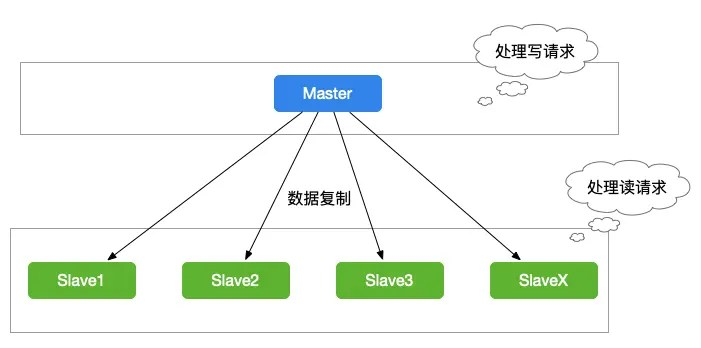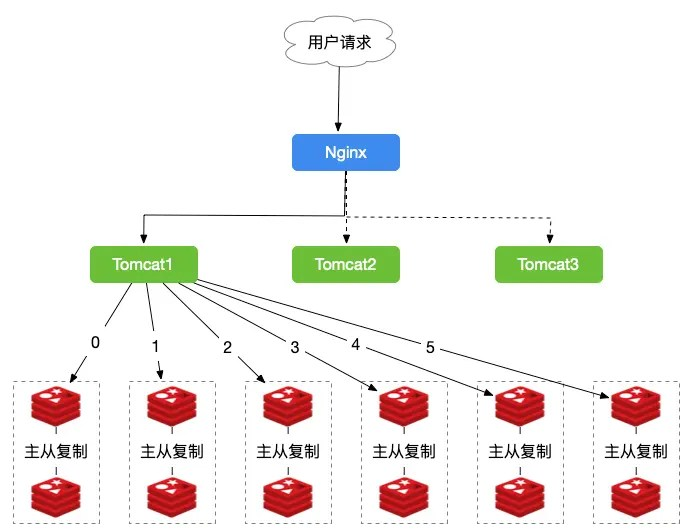### 使用Hash的Redis集群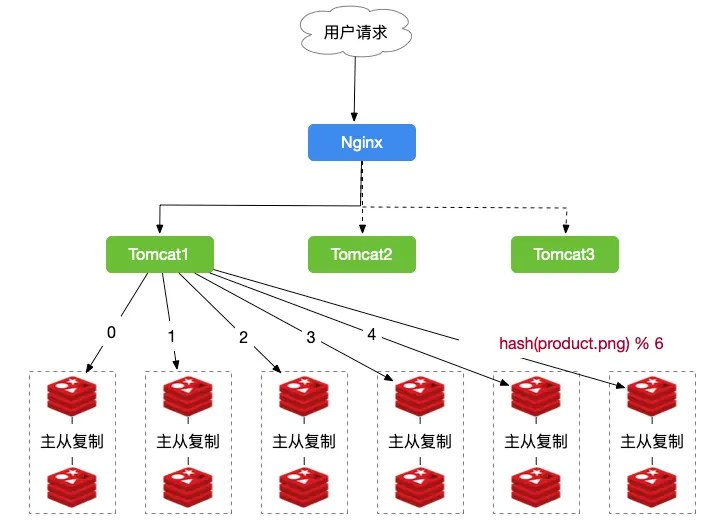### 一致性Hash算法原理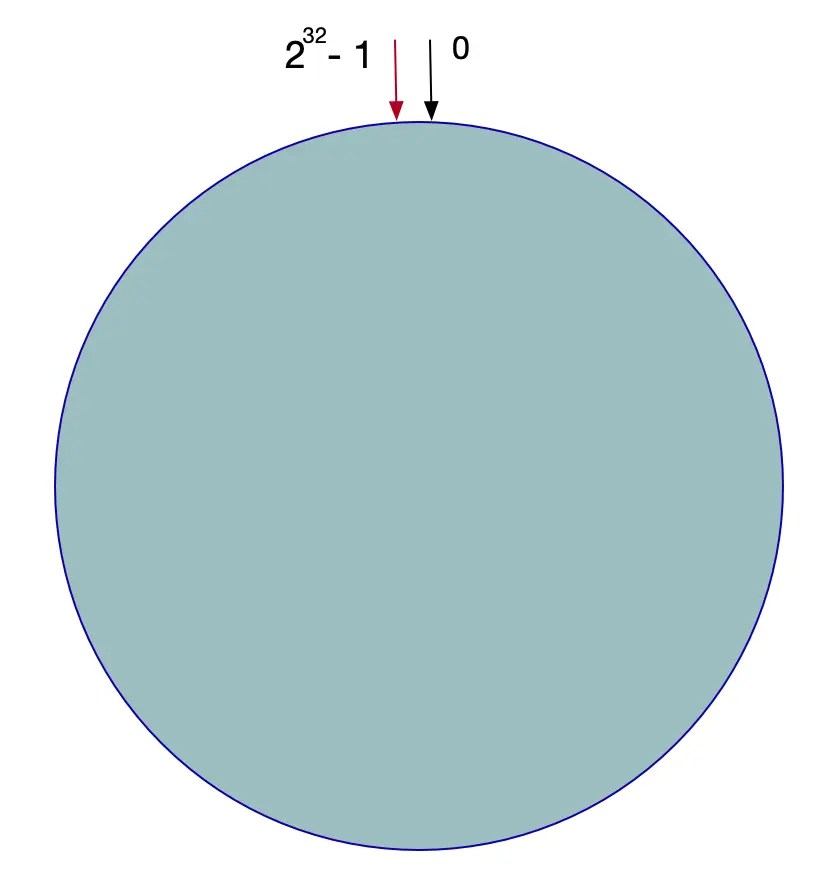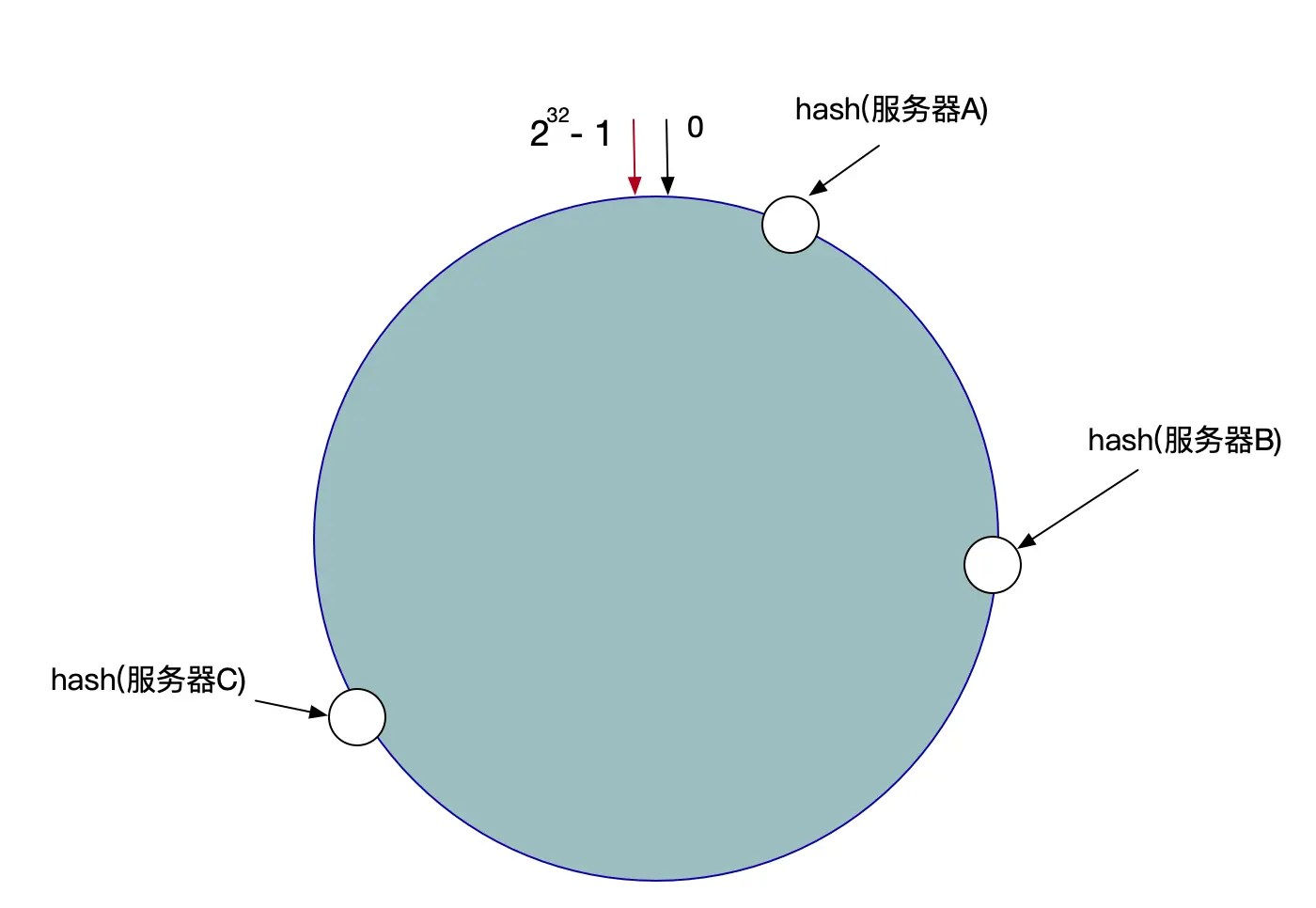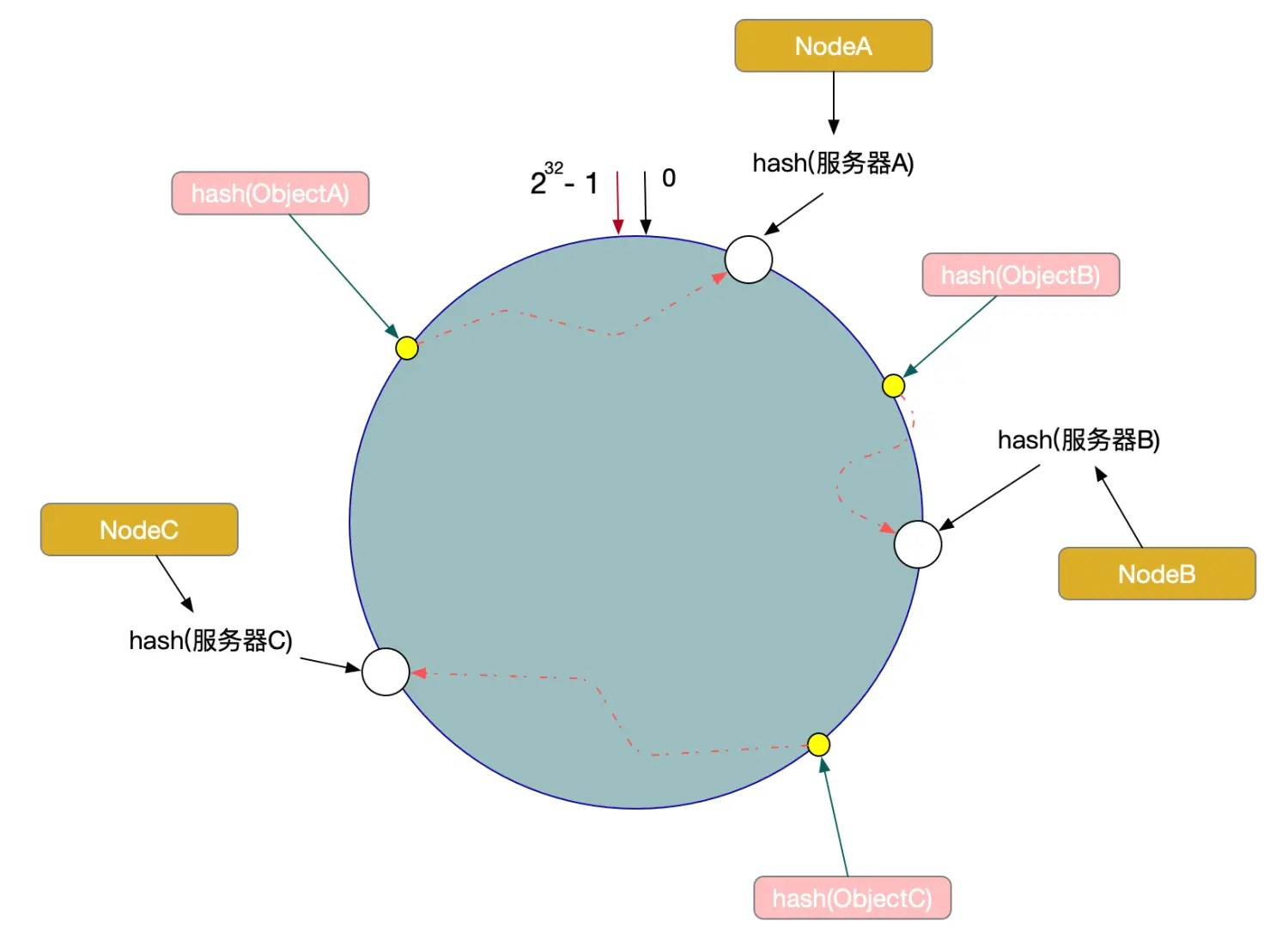### 一致性Hash算法的容错性和可扩展性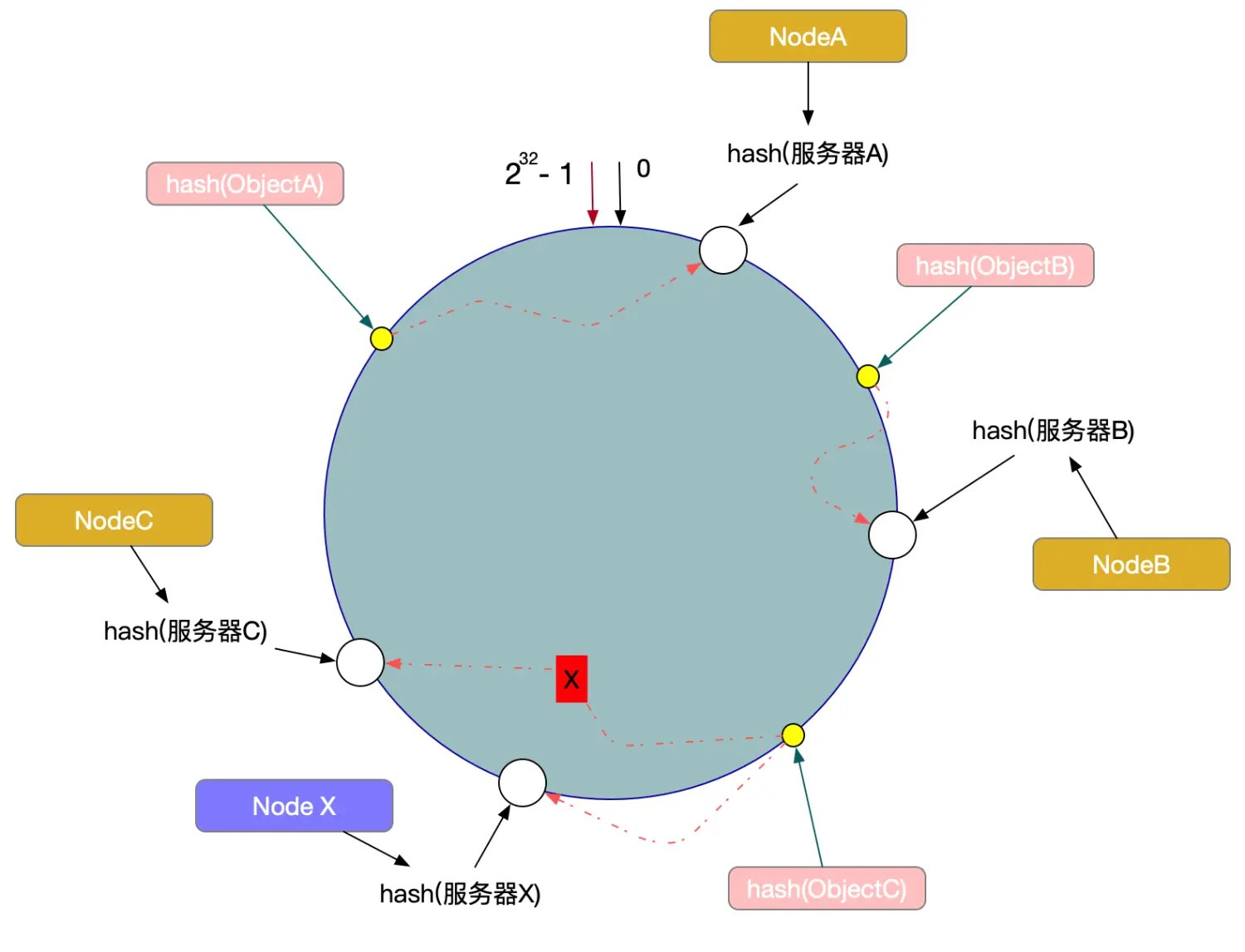### 数据倾斜问题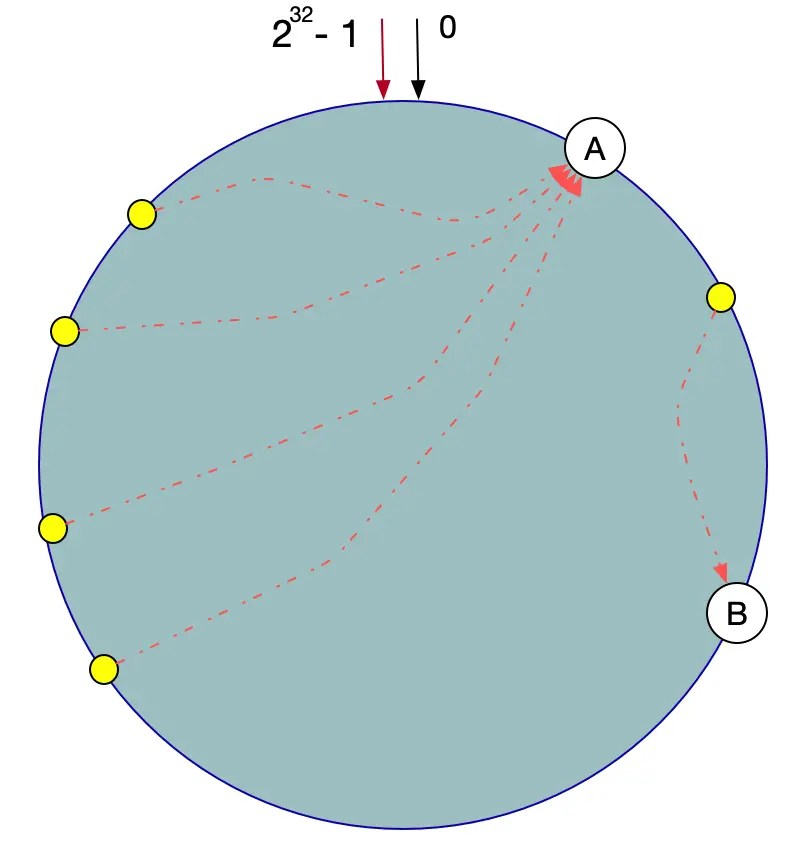### 具体实现

``````public interface IHashService {
Long hash(String key);
}
``````

``````public class HashService implements IHashService {

/**
* MurMurHash算法,性能高,碰撞率低
*
* @param key String
* @return Long
*/
public Long hash(String key) {
ByteBuffer buf = ByteBuffer.wrap(key.getBytes());
int seed = 0x1234ABCD;

ByteOrder byteOrder = buf.order();
buf.order(ByteOrder.LITTLE_ENDIAN);

long m = 0xc6a4a7935bd1e995L;
int r = 47;

long h = seed ^ (buf.remaining() * m);

long k;
while (buf.remaining() >= 8) {
k = buf.getLong();

k *= m;
k ^= k >>> r;
k *= m;

h ^= k;
h *= m;
}

if (buf.remaining() > 0) {
ByteBuffer finish = ByteBuffer.allocate(8).order(ByteOrder.LITTLE_ENDIAN);
finish.put(buf).rewind();
h ^= finish.getLong();
h *= m;
}

h ^= h >>> r;
h *= m;
h ^= h >>> r;

buf.order(byteOrder);
return h;

}
}
``````

``````public class Node<T> {
private String ip;
private String name;

public Node(String ip, String name) {
this.ip = ip;
this.name = name;
}

public String getIp() {
return ip;
}

public void setIp(String ip) {
this.ip = ip;
}

public String getName() {
return name;
}

public void setName(String name) {
this.name = name;
}

/**
* 使用IP当做hash的Key
*
* @return String
*/
@Override
public String toString() {
return ip;
}
}
``````

``````public class ConsistentHash<T> {
// Hash函数接口
private final IHashService iHashService;
// 每个机器节点关联的虚拟节点数量
private final int          numberOfReplicas;
// 环形虚拟节点
private final SortedMap<Long, T> circle = new TreeMap<Long, T>();

public ConsistentHash(IHashService iHashService, int numberOfReplicas, Collection<T> nodes) {
this.iHashService = iHashService;
this.numberOfReplicas = numberOfReplicas;
for (T node : nodes) {
}
}

/**
* 增加真实机器节点
*
* @param node T
*/
for (int i = 0; i < this.numberOfReplicas; i++) {
circle.put(this.iHashService.hash(node.toString() + i), node);
}
}

/**
* 删除真实机器节点
*
* @param node T
*/
public void remove(T node) {
for (int i = 0; i < this.numberOfReplicas; i++) {
circle.remove(this.iHashService.hash(node.toString() + i));
}
}

public T get(String key) {
if (circle.isEmpty()) return null;

long hash = iHashService.hash(key);

// 沿环的顺时针找到一个虚拟节点
if (!circle.containsKey(hash)) {
SortedMap<Long, T> tailMap = circle.tailMap(hash);
hash = tailMap.isEmpty() ? circle.firstKey() : tailMap.firstKey();
}
return circle.get(hash);
}
}
``````

``````public class TestHashCircle {
// 机器节点IP前缀
private static final String IP_PREFIX = "192.168.0.";

public static void main(String[] args) {
// 每台真实机器节点上保存的记录条数
Map<String, Integer> map = new HashMap<String, Integer>();

// 真实机器节点, 模拟10台
List<Node<String>> nodes = new ArrayList<Node<String>>();
for (int i = 1; i <= 10; i++) {
map.put(IP_PREFIX + i, 0); // 初始化记录
Node<String> node = new Node<String>(IP_PREFIX + i, "node" + i);
}

IHashService iHashService = new HashService();
// 每台真实机器引入100个虚拟节点
ConsistentHash<Node<String>> consistentHash = new ConsistentHash<Node<String>>(iHashService, 500, nodes);

// 将5000条记录尽可能均匀的存储到10台机器节点上
for (int i = 0; i < 5000; i++) {
// 产生随机一个字符串当做一条记录，可以是其它更复杂的业务对象,比如随机字符串相当于对象的业务唯一标识
String data = UUID.randomUUID().toString() + i;
// 通过记录找到真实机器节点
Node<String> node = consistentHash.get(data);
// 再这里可以能过其它工具将记录存储真实机器节点上，比如MemoryCache等
// ...
// 每台真实机器节点上保存的记录条数加1
map.put(node.getIp(), map.get(node.getIp()) + 1);
}

// 打印每台真实机器节点保存的记录条数
for (int i = 1; i <= 10; i++) {
System.out.println(IP_PREFIX + i + "节点记录条数：" + map.get(IP_PREFIX + i));
}
}
}
``````